# HSSlive: Plus One & Plus Two Notes & Solutions for Kerala State Board

## BSEB Class 9 Maths Chapter 7 Triangles Ex 7.2 Textbook Solutions PDF: Download Bihar Board STD 9th Maths Chapter 7 Triangles Ex 7.2 Book AnswersBSEB Class 9 Maths Chapter 7 Triangles Ex 7.2 Textbook Solutions PDF: Download Bihar Board STD 9th Maths Chapter 7 Triangles Ex 7.2 Book Answers

BSEB Class 9th Maths Chapter 7 Triangles Ex 7.2 Textbooks Solutions and answers for students are now available in pdf format. Bihar Board Class 9th Maths Chapter 7 Triangles Ex 7.2 Book answers and solutions are one of the most important study materials for any student. The Bihar Board Class 9th Maths Chapter 7 Triangles Ex 7.2 books are published by the Bihar Board Publishers. These Bihar Board Class 9th Maths Chapter 7 Triangles Ex 7.2 textbooks are prepared by a group of expert faculty members. Students can download these BSEB STD 9th Maths Chapter 7 Triangles Ex 7.2 book solutions pdf online from this page.

## Bihar Board Class 9th Maths Chapter 7 Triangles Ex 7.2 Books Solutions

 Board BSEB Materials Textbook Solutions/Guide Format DOC/PDF Class 9th Subject Maths Chapter 7 Triangles Ex 7.2 Chapters All Provider Hsslive

## How to download Bihar Board Class 9th Maths Chapter 7 Triangles Ex 7.2 Textbook Solutions Answers PDF Online?

2. Click on the Bihar Board Class 9th Maths Chapter 7 Triangles Ex 7.2 Answers.
3. Look for your Bihar Board STD 9th Maths Chapter 7 Triangles Ex 7.2 Textbooks PDF.
4. Now download or read the Bihar Board Class 9th Maths Chapter 7 Triangles Ex 7.2 Textbook Solutions for PDF Free.

Find below the list of all BSEB Class 9th Maths Chapter 7 Triangles Ex 7.2 Textbook Solutions for PDF’s for you to download and prepare for the upcoming exams:

## BSEB Bihar Board Class 9th Maths Solutions Chapter 7 Triangles Ex 7.2

Question 1.
In an isosceles triangle ABC, with AB = AC, the bisectors of ∠B and ∠C intersect each other at O. Join A to O. Show that:
(i) OB = OC
(ii) AO bisects ∠A.
Solution:
(i) In ∆ ABC, we haveAB = AC
⇒ ∠B = ∠C [∵ Angles opposite to equal sides are equal]
12∠B = 12∠C
⇒ ∠OBC = ∠OCB
[∵ OB and OC bisect ∠s B and C respectively]
∴ ∠OBC = 12∠B and ∠OCB = 12∠c
⇒ OB = OC … (2)
[∵ Sides opp. to equal ∠s are equal]

(ii) Now, in ∆s ABO and ACO, we have
AB = AC [Given]
∠OBC = ∠CCB [From (1)]
∆OB = OC . [From (2)]
∴ By SAS criterion of congruence, we have
∆ABO ≅ ∆ACO
⇒ ∠BAO = ∠CAO
[∵ Corresponding parts of congruent triangles are equal]
⇒ AO bisects ∠BAC.

Question 2.
In ∆ ABC, AD is the perpendicular bisector of BC (see figure). Show that ∆ ABC is an isosceles triangle in which AB = AC.Solution:
In ∆s ABD and ACD, we have
DB = DC [Given]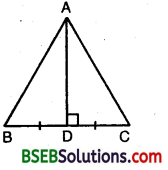∴ By SAS criterion of congruence, we have
∆ ABD ≅ ∆ ACD
⇒ AB = AC
[∵ Corresponding parts of congruent triangles are equal]
Hence, ∆ ABC is isosceles.

Question 3.
ABC is an isosceles triangle in Which altitudes BE and CF are drawn to sides AC and AB respectively (see figure). Show that these altitudes are equal.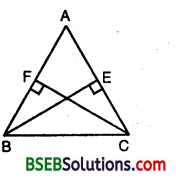Solution:
In ∆s ABE and ACF, we have
∠AEB = ∠AFC [∵ Each = 90°]
∠BAE = ∠CAF [Common]
and, BE = CF [Given]
By AAS criterion of congruence, we have
∆ ABE ≅ ∆ ACF
⇒ AB = AC
[∵ Corresponding parts of congruent triangles are equal]
Hence, ∆ ABC is isosceles.

Question 4.
ABC is a triangle in which altitudes BE and CF to sides AC and AB are equal (see figure). Show that
(i) ∆ ABE ≅ ∆ ACF
(ii) AB = AC, i.e., ABC is an isosceles triangle.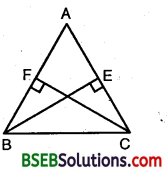Solution:
Let BE ⊥ AC and CF ⊥ AB.
In ∆s ABE and ACF, we have
∠AEB = ∠AFC [∵ Each = 90°]
∠A = ∠A [Common]
and, AB = AC [Given]
∴ By AAS criterion of congruence,
∆ ABE ≅ ∆ ACF
⇒ BE = CF
[∵ Corresponding parts of congruent triangles are equal]

Question 5.
ABC and DBC are two isosceles triangles on tile same base BC (see figure). Show that ∠ABD = ∠ACD.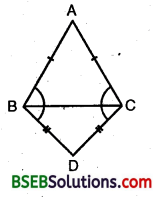Solution:
In ∆ ABC, we have
AB = AC
⇒ ∠ABC = ∠ACB
[∵ Angles opposite to equal sides are equal]
In ∆ BCD, we have
BO = CD
⇒ ∠DBC = ∠DCB … (2)
[∵ Angles opposite to equal sides are equal]
Adding (1) and (2), we have
∠ABC + ∠DBC = ∠ACB + ∠DCB
⇒ ∠ABD = ∠ACD

Question 6.
∆ ABC is an isosceles triangle in which AB = AC. Side BA is produced to D such that AD = AB (see figure). Show that ∠BCD is a right angle.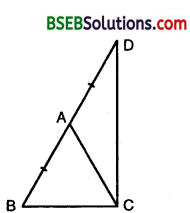Solution:
In ∆ ABC, we have
AB = AC
⇒ ∠ACB = ∠ABC … (1)
[∵ Angles opp. to equal sides are equal]
∴ AD = AC [∵AB = AC]
Thus, in ∆ ADC, we have
⇒ ∠ACD = ∠ADC … (2)
[∵ Angles opp. to equal sides are equal]
Adding (1) and (2), we get
∠ACB + ∠ACD = ∠ABC + ∠ADC
⇒ ∠BCD = ∠ABC + ∠BDC [∵ ∠ADC = ∠BDC]
⇒ ∠BCD + ∠BCD = ∠ABC + ∠BDC + ∠BCD [Adding ∠BCD on both sides]
⇒ 2∠BCD = 180°
⇒ ∠BCD = 90°
Hence, ∠BCD is a right angle.

Question 7.
ABC is a right angled triangle in which ∠A = 90 and AB = AC. Find ∠B and ∠C.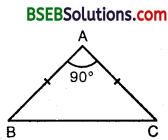Solution: We have, ∠A = 90°
AB = AC
⇒ ∠B = ∠C
[∵ Angles opp. to equal sides of a triangle are equal]
Also, ∠A + ∠B + ∠C = 180° [Angle-sum property]
⇒ 90° + 2∠B = 180° [∵ ∠C = ∠B]
⇒ 2∠B = 180° – 90° = 90°
⇒ ∠B = 90°2 = 45°
∴ ∠C = ∠B = 45°

Question 8.
Show that the angles of an equilateral triangle are 60° each.
Solution:
Let ∆ ABC be an equilateral triangle so that AB = AC = BC.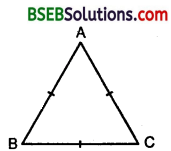Now, ∵ AB = AC
⇒ ∠B = ∠C … (1)
[∵ Angles opp. to equal sides are equal]
Also, ∵ CB = CA
⇒ ∠A = ∠B … (2)
[∵ Angles opp. to equal sides are equal]
From (1) and (2), we have
∠A = ∠B = ∠C
Also, ∠A + ∠B + ∠C = 180° [Angle-sum property]
∴ ∠A + ∠A + ∠A = 180°
⇒ 3∠A = 180° ⇒ ∠A = 60°
∴ ∠A = ∠B = ∠C = 60°
Thus, each angle of an equilateral triangle is 60°.

## Bihar Board Class 9th Maths Chapter 7 Triangles Ex 7.2 Textbooks for Exam Preparations

Bihar Board Class 9th Maths Chapter 7 Triangles Ex 7.2 Textbook Solutions can be of great help in your Bihar Board Class 9th Maths Chapter 7 Triangles Ex 7.2 exam preparation. The BSEB STD 9th Maths Chapter 7 Triangles Ex 7.2 Textbooks study material, used with the English medium textbooks, can help you complete the entire Class 9th Maths Chapter 7 Triangles Ex 7.2 Books State Board syllabus with maximum efficiency.

## FAQs Regarding Bihar Board Class 9th Maths Chapter 7 Triangles Ex 7.2 Textbook Solutions

#### Can we get a Bihar Board Book PDF for all Classes?

Yes you can get Bihar Board Text Book PDF for all classes using the links provided in the above article.

## Important Terms

Bihar Board Class 9th Maths Chapter 7 Triangles Ex 7.2, BSEB Class 9th Maths Chapter 7 Triangles Ex 7.2 Textbooks, Bihar Board Class 9th Maths Chapter 7 Triangles Ex 7.2, Bihar Board Class 9th Maths Chapter 7 Triangles Ex 7.2 Textbook solutions, BSEB Class 9th Maths Chapter 7 Triangles Ex 7.2 Textbooks Solutions, Bihar Board STD 9th Maths Chapter 7 Triangles Ex 7.2, BSEB STD 9th Maths Chapter 7 Triangles Ex 7.2 Textbooks, Bihar Board STD 9th Maths Chapter 7 Triangles Ex 7.2, Bihar Board STD 9th Maths Chapter 7 Triangles Ex 7.2 Textbook solutions, BSEB STD 9th Maths Chapter 7 Triangles Ex 7.2 Textbooks Solutions,
Share: